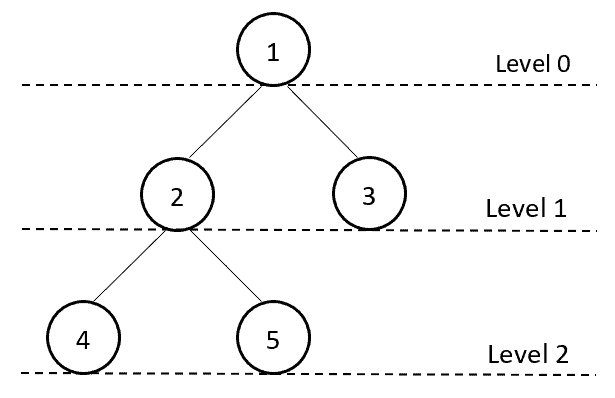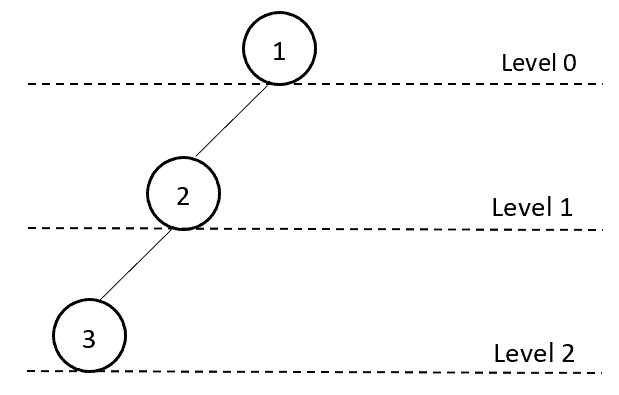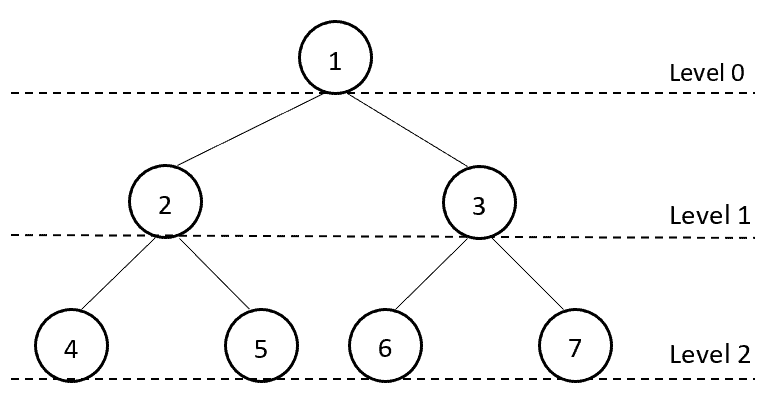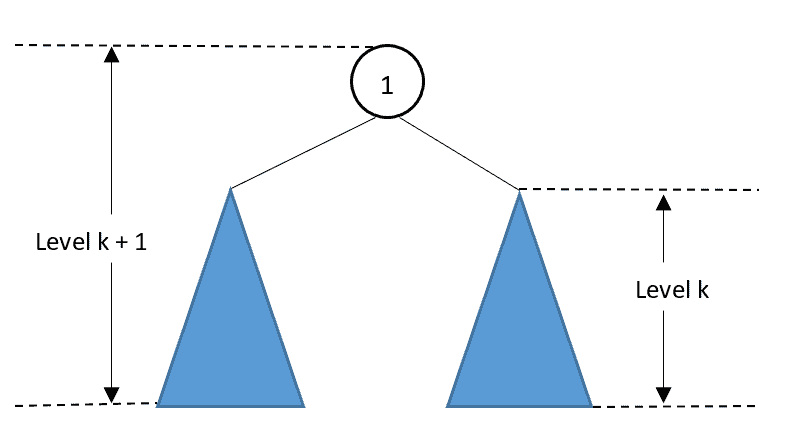## 1. Introduction

A binary tree is a hierarchical data structure in which each node has at most two children. This tutorial will show how to calculate the binary tree level and the number of nodes. We’ll also examine the relationship between the tree level and the number of nodes.

## 2. Binary Tree Level

In a binary tree, each node has 3 elements: a data element to hold a data value, and two children pointers to point its left and right children:The topmost node of a binary tree is the root node. The level of a node is the number of edges along the unique path between it and the root node. Therefore, the root node has a level of 0. If it has children, both of them have a level of 1.

The level of a binary tree is the highest level number among all nodes. For example, we can use 5 nodes to construct a binary tree with a level number of 2:## 3. Tree Level and Number of Nodes Calculation

To calculate the level of a binary tree, we can traverse the tree level-by-level. We start with the root node as level 0. Then we visit every node on a level before going to another level. For example, the level-by-level traversal sequence of the above example tree is 1, 2, 3, 4, 5.

The level-by-level binary tree traversal is also a breadth-first search (BFS) approach. To achieve this traversal, we can use a queue data structure to ensure the order of traversal.

Each element of the queue contains two values, the current tree node and the level number of the current tree node:After the traversal, the maximum level number will be the tree’s level number. We can also use a counter to count number of nodes during the traversal:In this algorithm, we start with thenode. First, we put thenode and its level number into the queue. Then we use a loop to traverse all the tree nodes by their levels.

In each iteration, we increase the current tree node’s level by 1 and associate it with the node’s left and right children. During the traversal, we record the maximum level number. After we visit all the tree nodes, the maximum value is then the binary tree’s level.

If the binary tree containsnodes, the overall run time of this algorithm issince we visit each node only once.

## 4. Minimum and Maximum Number of Nodes

We can use the algorithm above to calculate the exact number of nodes of a binary tree and its level number. Furthermore, we can determine the minimum and a maximum number of nodes for a binary tree with levelwith theoretical analysis.

### 4.1. Minimum Number of Nodes

It’s easy to see that we need at least one node for each level to construct a binary tree with level. Therefore, the minimum number of nodes of a binary tree with levelis. This binary tree behaves like a linked list data structure:We can conclude the minimum number of nodes with the following theorem:### 4.2. Maximum Number of Nodes

To construct a binary tree of levelwith the maximum number of nodes, we need to make sure all the internal nodes have two children. In addition, all the leaf nodes must be at the level.

For example, at level 0, we only have the root node. At level 1, we have 2 nodes that are the children of the root. Similarly, we have 4 nodes at level 2 who are the children of the nodes in level 1:Based on this observation, we can see that each level doubles the number of nodes from its previous level. This is because every internal node has two children. Therefore, the maximum number of nodes of a levelbinary tree is. We also call this type of binary tree a full binary tree.

We can conclude the maximum number of nodes with the following theorem:## 5. Proof by Induction

We can also use induction to prove Theorem 2. For the base case, where, a binary tree of level 0 is just a single root node without any children. Also, when, we have. Therefore, the base case satisfies the induction hypothesis:In the induction step, letbe a binary tree of level. For the root node of, its right subtree and left subtree both have level:The total number of nodes ofis the sum of its two subtrees and the root node. Therefore,contains at mostnodes. The hypothesis holds for, and so the theorem is proved.

## 6. Conclusion

This article showed how to calculate the level and number of nodes for a binary tree. We also provided theoretical results on the minimum and the maximum number of nodes for a binary tree of level. In the end, we used induction to prove that the maximum number of nodes for a levelbinary tree is.